American Journal of Applied Mathematics
Volume 3, Issue 5, October 2015, Pages: 221-228

Friction Coefficient’s Numerical Determination for Hot Flat Steel Rolling at Low Strain Rate

1Department of Mechanical Engineering, University of Agriculture, P. M. B., Abeokuta, Nigeria

2Department of Mechanical Engineering, University of Lagos, Lagos, Nigeria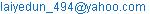(P. Aiyedun)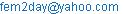(A.O. Oni)

Peter Aiyedun, O. Ogunlade, A. O. Oni, Olayide Adetunji. Friction Coefficient’s Numerical Determination for Hot Flat Steel Rolling at Low Strain Rate. American Journal of Applied Mathematics.Vol.3, No. 5, 2015, pp. 221-228. doi: 10.11648/j.ajam.20150305.13

Abstract: In this paper, a non-linear quadratic expression of the coefficient of friction at both entry and exit sides have been derived from the equations of rolling load in Hot Rolling Bland and Ford’s program (HRBF). The study developed a numerical model for the estimation of coefficient of friction for steel (HC SS316) of different thicknesses on two high reversing mills. The equations for coefficient of friction on entry and exist sides of the mills were modelled from Hot Rolling Bland and Ford’s program (HRBF). The equations were modelled such that the friction coefficient can be expressed as a function of process parameters measurable during rolling. The capability of the model was verified by using a number of specimens of HC SS316 with predetermined hot rolling experimental data. A good agreement was noted between the predicted friction coefficient and the measured one.

Keywords: Rolling Mill A and B, Coefficient of Friction, Strain Rate

1. Introduction

The effects of friction in rolling operations have always been an important topic of interest. This is due to the fact that friction significantly affects the quality of materials and the processing parameters. For this reason, frictional effects are considered to be a major variable that must be controlled in order to optimize processing procedures to achieve economic goals without impairing surface quality, the desired geometry or internal structure of the product. Therefore, an accurate evaluation of the friction conditions during rolling operations is required as a quality control measure.

The friction coefficient is regarded to be one of the most important parameters in rolling processes (Altınkaya et al., 2014). Different methods for the predictions of coefficient of friction have been widely reported. The most common approach is to evaluate coefficient of friction from one or more rolling parameters. This is because the direct measurement of friction coefficient is a difficult procedure (Gudur et al., 2008; Tan et al., 2008). The early works of Ekelund (1933), Wusatowski (1969) and Sparling (1961) involved empirical models by examining rolling parameters from the observed experimental investigations data. The notable theoretical models for the evaluation coefficient of friction include homogeneous and inhomogeneous deformation equations. The theory of homogeneous deformation equations was introduced by Karman (1925). This theoretical technique was established from a consideration of equilibrium of forces acting on the elemental slab in the zone of deformation. The principal assumption is that the deformation of materials is homogenous throughout the whole deformation zone. However, the theoretical results generally show poor agreement with experimental results (Jing, 2001). Orowan (1943) in his theory of inhomogenous deformation assumed that stress distribution in a vertical plane is not homogenous. He obtained a more accurate result. However, Sims (1954) improved on Orowan’s original method but with the assumption that sticking friction exists along the arc of contact in hot rolling. A good agreement was shown between the results of experiment and calculation. Furthermore, Chen et al. (2014) also improved Karman equation for hot-rolled strip. A new rolling pressure formula was deduced based on comprehensive consideration of the slipping and sticking friction on the contact arc between hot-rolled strip and work rolls. Their work also analysed the influence of friction condition, flow stress, roller distortion and other factors on the results of rolling pressure and force per unit width. The computational results demonstrate that the proposed new model improves the setting precision of roll force and can be applied to online control of hot rolled strip. The introduction and application of other theoretical methods such as slip line analysis (Alexander, 1955), the upper bound theory (Siebel, 1925; Johnson and Kudo, 1960) and finite element method (Hwang and Joun, 1992; Moon and Lee, 2008; Wang et al., 2010; Zhang and Cui, 2011) have been used for the analysis of coefficient of friction in metal rolling operations. However, these theoretical methods has not been able to provide detailed behaviour of work piece thus coefficient of friction (Ginzburg, 1989; Robert, 1983). Each theoretical method has its own merits and limitations.

Although many investigators are working on rolling and many prints are available (Legrand et al., 2012; Zhang et al., 2012; Parvizi and Abrinia, 2014), some fields remain practically unexplored. Since this is an expanding field, there is still much work that needs to be done on rolling design. In this work, The Bland and Ford’s approach where slipping friction exist throughout the roll gap using Orowon’s general equation of flat rolling, is also used to numerically determine the coefficient of friction for hot flat rolling of steel at low strain rate. In order to determine the coefficient of friction, a quadratic expression of the coefficient at both entry and exit sides were generated from the Hot Rolling Bland and Ford’s program (HRBF) of Aiyedun (1984) by making it the subject of formula from the equations of the rolling load through mathematical analysis. MATLAB computer algorithm was then used to develop a computer code for the coefficient of friction equation using sequential quadratic programming method.

2. Mathematical Model

2.1. Determination of Coefficient of Friction from Hot Rolling Bland and Ford’s (HRBF) Program

The mathematical model used for the simulation is derived from the rolling load (FP) of Hot Rolling Bland and Ford’s (HRBF) program.

2.1.1. Coefficient of Friction at the Entry Zone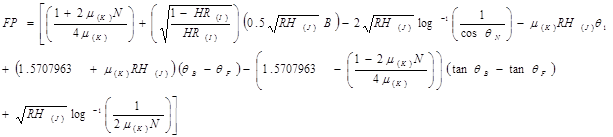(1)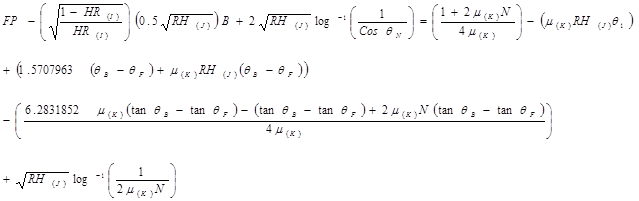(2)

We multiply both sides by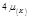and expand the expression, we have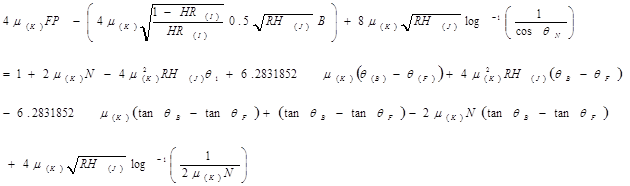(3)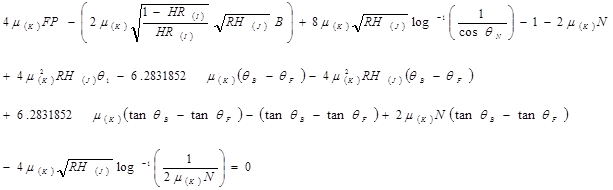(4)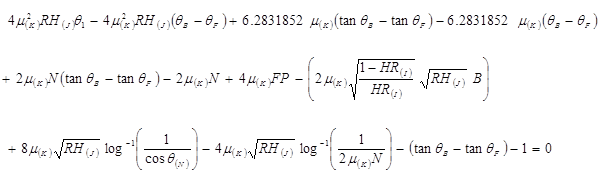(5)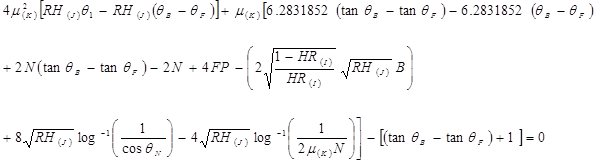(6)

This expression for µ in the equation above is a complex non-linear quadratic equation which can only be solved by a numerical method.

From El-Kalay , the above expression can further be expressed as: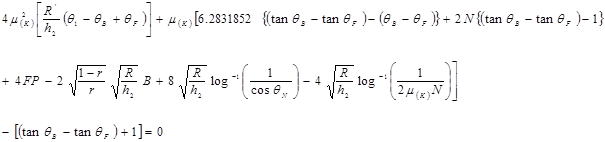(7)

2.1.2. Coefficient of Friction at the Exit Zone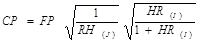(8)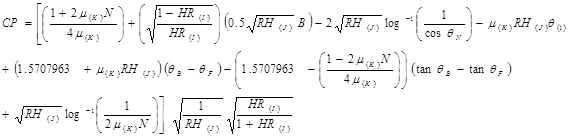(9)

We multiply through by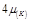and expand the expression, we have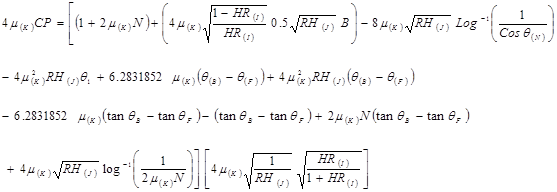(10)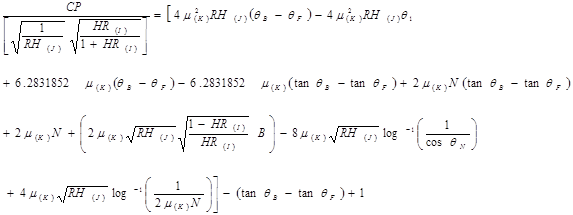(11)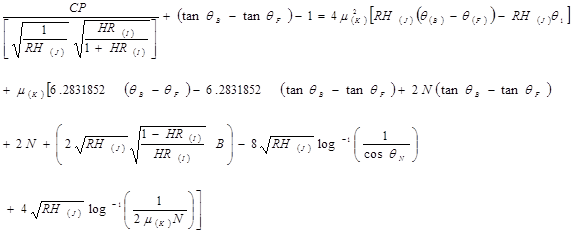(12)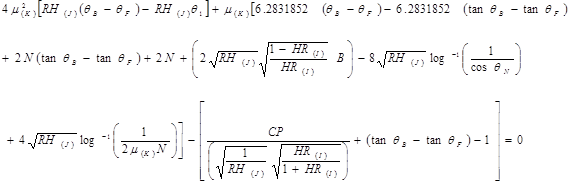(13)

but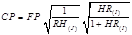in equation (8) and substituting into equation (13),

we have:-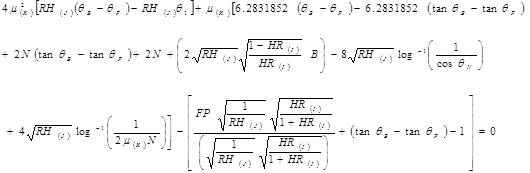(14)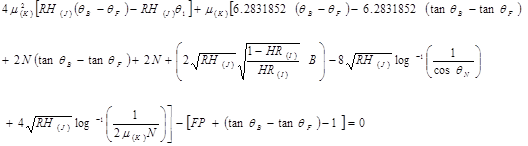(15)

Also, from El-Kalay (1966), the above expression can further be expressed as;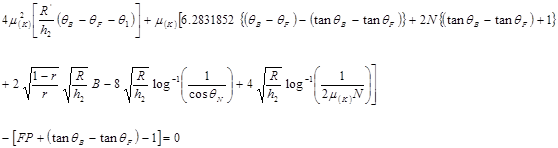(16)

2.2. Model Simulation and Validation

The equation of the rolling load (FP) was retrieved from Hot Rolling Bland and Ford’s program in which a quadratic expression for the coefficient of friction was then generated. The computation of the coefficient of friction equation was integrated into the Sequential Quadratic Programming (SQP) method, which is a numerical method, and was run on MATLAB computer software.

Validation of the computer codes was then carried out using a number of specimens of HC SS316 with predetermined hot rolling experimental data from Tables 4.1 and 4.2 of Aiyedun (1984). These specimens with different initial thickness were hot rolled at varying low reductions, widths, furnace temperatures, rolling speed etc. on two-high reversing rolling mill A (Specimens H20-H57) and B (Specimens P30-P65).

3. Results and Discussion

The computed results of the coefficient of friction (µ) at the entry and exit sides against the rolling conditions (reduction (%) and equivalent strain rate) are illustrated graphically in Figs. 1 - 4. A comparison between Tamano and Yanagimoto (1970) and the present work is presented in Fig. 5.

3.1. Effect of the Rolling Conditions on the Coefficient of Friction (µ) on the Entry and Exit Sides

Fig. 1 shows the effect of reduction (%) on the coefficient of friction for HC SS316 on specimens rolled on mill A (H32-H35 and H36-H44) on the entry and exit sides. It was observed that as the percentage reduction increased, the coefficient of friction decreases accordingly to a minimum value before increasing, that of the exit side being more pronounced.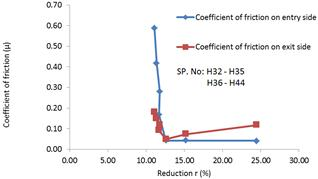Fig. 1. Effect of Reduction, r (%) on the Coefficient of friction for HC SS316 on Specimens rolled on Mill A on the entry and exit sides.

Fig. 2 shows the effect of reduction (%) on the coefficient of friction for HC SS316 on specimens rolled on mill B (P41-P44 and P47-P53) on the entry and exit side. It was observed that as the percentage reduction decreased before increasing, the coefficient of friction on both the entry and exit side first decreases to a minimum value before increasing, that of the exit side being more pronounced.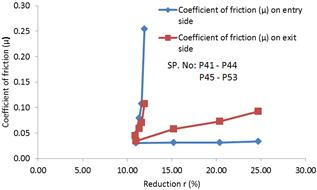Fig. 2. Effect of Reduction, r (%) on the Coefficient of friction for HC SS316 on Specimens rolled on Mill B on the entry and exit sides.

Fig. 3 shows the effect of equivalent strain rate on the coefficient of friction for HC SS316 at low reduction on specimen rolled on mill A (H32-H35 and H36-H44) on the entry and exit sides. It was observed that as the strain rate increased, the coefficient of friction on both the entry and exit sides first decreased to a minimum value before increasing on the exit side.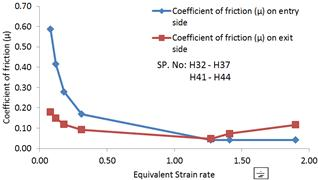Fig. 3. Effect of Equivalent Strain rate on the Coefficient of friction for HC SS316 at low reduction, on Specimens rolled on Mill A on the entry and exit sides.

Fig. 4 shows the effect of equivalent strain rate on the coefficient of friction for HC SS316 at low reduction on specimen rolled on mill B (P41-P44 and P47-P53) on the entry and exit side. It was observed that as the strain rate increased, the coefficient of friction on both the entry and exit side first decreased uniformly to a minimum value before increasing that of the exit side being more pronounced.Fig. 4. Effect of Equivalent Strain rate on the Coefficient of friction for HC SS316 at low reduction, on Specimens rolled on Mill B on the entry and exit sides.

3.2. Comparison of the Present Work with (Tamano and Yanagimoto 1970)

The solution in this work at the entry side is compared with Tamano and Yanagimoto (1970). It is natural that there should be a great difference between the present solution and Tamano and Yanagimoto (1970) because of the difference in the state of friction. Although, the present solution deals with friction coefficient in the roll gap for various specimens with varying and approximately the same percentage reduction whereas Tamano and Yanagimoto (1970) addresses the situation of rolling in the whole state of friction. This encompasses slipping friction, mixed friction or sticking friction which depend on geometrical and physical rolling factors (viz. radius of roll, thickness of strip before and after rolling, rolling temperature, yield stress of materials, and surface and lubricating condition of roll and material). Both the coefficient of friction in the two solutions tends to decrease as the percentage reduction increased, and as the strain rate increased.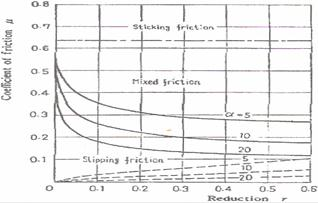Fig. 5. Relation between the rolling conditions and the state of friction, after Tamano and Yanagimoto (1970).

4. Conclusions

In this paper, a non-linear quadratic expression of the coefficient of friction at both entry and exit sides have been derived from the equations of rolling load in Hot Rolling Bland and Ford’s program (HRBF) of Aiyedun (1984).

From the present theoretical investigation, the following conclusions were drawn:

i.        Bland and Ford’s approach gave a satisfactory result for the estimation of the coefficient of friction in hot flat rolling of steel (HC SS316) at low strain rate when compared with the solution found in Tamano and Yanagimoto (1970).

ii.       For specimen rolled on mill A (H32-H35 and H36-H44) on the entry and exit sides, the effect of reduction (%) on the coefficient of friction (µ) for HC SS316 shows that, as the percentage reduction increased, the coefficient of friction (µ) decreases accordingly before increasing, that of the exit side being more pronounced.

iii.       For specimen rolled on mill B (P41-P44 and P47-P53) on the entry and exit sides, the effect of reduction (%) on the coefficient of friction (µ) for HC SS316 shows that, as the percentage reduction decreased before increasing, the coefficient of friction (µ) first decreases to a minimum value before increasing, that of the exit side being more pronounced.

iv.       For specimen rolled on mill A (H32-H35 and H36- H44) on the entry and exit sides, the effect of equivalent strain rate on the coefficient of friction (µ) for HC SS316 at low reduction, shows that, as the strain rate increased, the coefficient of friction (µ) first decreased to a minimum value before increasing, that of the exit side being more pronounced.

v.        For specimen rolled on mill B (P41-P44 and P47-P53) on the entry and exit sides, the effect of equivalent strain rate on the coefficient of friction (µ) for HC SS316 at low reduction, shows that, as the strain rate increased, the coefficient of friction (µ) first decreased to a minimum value before increasing, that of the exit side being more pronounced.

vi.       When the present work was compared with the solutions in Tamano and Yanagimoto (1970), there was a great difference in the state of friction. The present solution deals with friction coefficient in the roll gap for various specimens with varying and approximately the same percentage reduction while Tamano and Yanagimoto (1970) addresses the situation of rolling in the whole state of friction (slipping friction, mixed friction or sticking friction) which depend on geometrical and physical rolling factors (radius of roll, thickness of strip before and after rolling, rolling temperature, yield stress of materials, and surface and lubricating condition of roll and material). Both the coefficient of friction in the two solutions tends to decrease as the percentage reduction increased, and as the strain rate increased.

vii.       At low strain rate (0.08-1.5s-1), the coefficient of friction decreases uniformly at the whole roll gap for the range of specimens observed.

Nomenclature

h1 = thickness before rolling (mm);

h2 = thickness after rolling (mm)

hx = thickness of material at some point in the roll gap.

S = specific normal pressure (N/mm2)

P = vertical roll pressure (N/mm2)

Q = horizontal compressive stress in the material at point of thickness h; at hx+dhx, stress = σx+dσx

R = roll radius (undeformed) (mm)

R’ = elastically deformed roll radius (mm)

q = angle subtended by a point on roll surface at center of curvature of arc of contact with respect to line joining roll centers

fN = angle subtended by neutral plane with respect to vertical

a = angle of entry (i.e. maximum value of q) in rad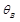= angle at which sticking starts in hot rolling bland & ford’s approach (radians)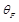= angle at which sticking ends in hot rolling bland & ford’s approach (radians)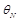= neutral angle (radians)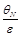= equivalent strain rate(s-1)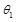= angle of contact in hot rolling bland & ford’s approach (radians)

H = Bland & Ford’s function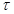= Frictional stress acting on the roll surface, kg/mm2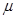= Coefficient of friction

B = width of Strip (mm)

D = Diameter of Roll cylinder specimen (mm)

Δh = rolling draught

V1 = entry velocity (mm)

V2 = exit velocity (mm)

σ = flow stress of the material in plane compression (kg/mm2)

α = geometrical factor

r = reduction, expressed as a fraction

C = integration constant

Suffixes

1 - conditions at exit plane

2 refers to conditions at entry plane

n refers to conditions at the neutral plane

f refers to conditions at the frictional transition plane on the exit side

b refers to conditions at the frictional transition plane on the entry side

References

1. Altınkaya, H. Orak, I.M. Esen. I. (2014). Artificial neural network application for modeling the rail rolling process. Expert Systems with Applications 41: 7135–7146.
2. Alexander, J.M. (1972). Proc. R. Soc., A326, 535-563.
3. Aiyedun, P.O. (1984). A Study of Loads and Torque for Light Reduction in Hot Flat Rolling at Low strain rates. PhD. Thesis. University of Sheffield, UK.
4. Chen, S. Li, W. Liu, X. (2014). Calculation of rolling pressure distribution and force based on improved Karman equation for hot strip mill. International Journal of Mechanical Sciences 89 256–263.
5. Ekelund, S. (1933). Steel, 93, Weekly issues for 21 Aug. to 2 Oct 1933.
6. El-Kalay, K.E.H.A (1966). A study of the factors affecting friction and their effects upon load,
7. Torque and spread in hot flat rolling. PhD. Thesis, University of Sheffield, UK.
8. Ginzburg, V. B. (1989). Steel-rolling Technology, Pittsburgh, Pennsylvania.
9. Gudur, P.P. Salunkhe, M.A. Dixit, U.S. (2008). A theoretical study on the application of asymmetric rolling for the estimation of friction. International Journal of Mechanical Sciences 50: 315–327.
10. Hwang, S.M. Joun, M.S. (1992). Analysis of hot-strip rolling by a penalty rigid-viscoplastic finite element method, Int. J. Mech. Sci. 34: 971–984.
11. Jing, L. (2001). Rolling mill roll design, Durhan thesis, Durham University. www.ethesis.dur.ac.uk/3959/
12. Johnson, W. and Kudo, H. (1960). Int. J. Mech. Sci., 1, 175-191.
13. Karman, V (1925). Th., angew, Z. Math. Mech., 5, 139-141.
14. Legrand, N. Lavalard, T. Martins, A. (2012). New concept of friction sensor for strip rolling: Theoretical analysis. Wear 286– 287 (2012) 8 – 18.
15. Moon, C.H. Lee, Y. (2008). Approximate model for predicting roll force and torque in plate rolling with peening effect considered, ISIJ Int. 48: 1409–1418.
16. Orowan, E. (1943). Proc. Inst. Mech.Eng. 150, 140-167.
17. Parvizi, A. Abrinia, K. (2014). A two dimensional upper bound analysis of the ring rolling process with experimental and FEM verifications. International Journal of Mechanical Sciences 79: 176–181.
18. Roberts, W. L. (1988). Flat Processing of Steel, Dekker, N York.
19. Siebel, E. (1925). Eisen, Stahl. 45, 1563-1566.
20. Sims, R. B. (1954). Proc, Inst. Mech. Eng.168, 191-200.
21. Sparling, L. M. G. (1961). Formula for spread in Hot Flat Rolling, Proc. Int. Mech. Eng., Vol. 175, No.ll, pp. 604-640.
22. Tan, X. Yan, X. Juster, N.P. Raghunathan S. Wang, J. (2008). Dynamic friction model and its application in flat rolling. Journal of materials processing technology 207: 222–234.
23. Tamano, T. and Yanagimoto, S. (1970). Theory of rolling for the Range of mixed Friction", Bulletin of the JSME, 13: 63: 1134-1141.
24. Wang, X. Peng, Y. Xu, L. Liu, H. (2010). A 3-D differential method for solving rolling force of PC hot strip mill, J. Iron Steel Res. Int. 17: 36–39.
25. Wusatowski, Z. (1969). Fundamentals of Rolling Gliwice, Poland.
26. Zhang, J. Cui, Z. (2011). Continuous FEM simulation of multi-pass plate hot rolling suitable for plate shape analysis, J. Cent. South Univ. Technol. 18 (2011) 16–22.
27. Zhang, S.H. Zhao, D.W. Gao, C.R. Wang G.D. (2012). Analysis of asymmetrical sheet rolling by slab method. International Journal of Mechanical Sciences 65: 168–176.

 Contents 1. 2. 2.1. 2.2. 3. 3.1. 3.2. 4.
Article ToolsAbstractPDF(714K)